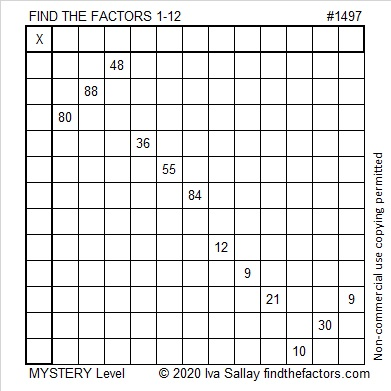# 1497 Mystery

Contents

### Today’s Puzzle:

Knowing where to place some of the numbers from 1 to 12 in this puzzle shouldn’t be too difficult, but placing ALL of the numbers will be like solving a mystery.### Factors of 1497:

• 1497 is a composite number.
• Prime factorization: 1497 = 3 × 499
• 1497 has no exponents greater than 1 in its prime factorization, so √1497 cannot be simplified.
• The exponents in the prime factorization are 1, and 1. Adding one to each exponent and multiplying we get (1 + 1)(1 + 1) = 2 × 2 = 4. Therefore 1497 has exactly 4 factors.
• The factors of 1497 are outlined with their factor pair partners in the graphic below.### More about the Number 1497:

1497 is the difference of two squares in two different ways:
749² – 748² = 1497
251² – 248² = 1497

This site uses Akismet to reduce spam. Learn how your comment data is processed.